# Credit Risk Classifications¶

by: Keith Qu

Using the data set from Kaggle's Give Me Some Credit competition, which contains 150,000 observations with 10 features and the objective of predicting whether a lendee will have a serious delinquincy (90+ days past due) within 2 years.

Using the methods described, we were able to attain an AUC score of 0.866752 on the private leaderboard.

Contents:

In :
import numpy as np
import pandas as pd
import matplotlib.pyplot as plt
import seaborn as sns
from sklearn.manifold import TSNE
from scipy.stats import randint, uniform
from scipy import linalg
from sklearn.decomposition import PCA
from sklearn.preprocessing import MinMaxScaler, StandardScaler
from sklearn.ensemble import GradientBoostingClassifier, GradientBoostingRegressor, RandomForestClassifier
from sklearn.linear_model import LogisticRegression
from sklearn.model_selection import train_test_split, cross_val_predict, RandomizedSearchCV
from sklearn.metrics import classification_report, confusion_matrix, roc_auc_score
from sklearn.metrics import accuracy_score, r2_score, mean_squared_error
import xgboost as xgb
import random
%matplotlib inline


## Exploration, Cleaning, Creating Features¶

We have the following variables:

• SeriousDlqin2yrs (target): individual experiences a 90+ day overdue payment within 2 years
• age
• RevolvingUtilizationOfUnsecuredLines: balance on credit cards and lines of credit / sum of limits (excluding real etate, installment debt)
• DebtRatio: monthly debt, alimony, living cost payments divided by monthly income
• NumberRealEstateLoansOrLines: only real estate loans
• NumberOfOpenCreditLinesAndLoans: # install ment loans and LOCs
• NumberOfTime30-59DaysPastDueNotWorse: number of times person experienced 30-59 day lateness, but not more than that
• NumberOfTime60-89DaysPastDueNotWorse: same as above with 60-89 days
• NumberOfTime90DaysPastDueNotWorse: same as above with 90+
• NumberOfDependents: number of family members excluding self
• MonthlyIncome
In :
df = pd.read_csv('cs-training.csv',index_col=0)
df.head()

Out:
SeriousDlqin2yrs RevolvingUtilizationOfUnsecuredLines age NumberOfTime30-59DaysPastDueNotWorse DebtRatio MonthlyIncome NumberOfOpenCreditLinesAndLoans NumberOfTimes90DaysLate NumberRealEstateLoansOrLines NumberOfTime60-89DaysPastDueNotWorse NumberOfDependents
1 1 0.766127 45 2 0.802982 9120.0 13 0 6 0 2.0
2 0 0.957151 40 0 0.121876 2600.0 4 0 0 0 1.0
3 0 0.658180 38 1 0.085113 3042.0 2 1 0 0 0.0
4 0 0.233810 30 0 0.036050 3300.0 5 0 0 0 0.0
5 0 0.907239 49 1 0.024926 63588.0 7 0 1 0 0.0
In :
df.info()

<class 'pandas.core.frame.DataFrame'>
Int64Index: 150000 entries, 1 to 150000
Data columns (total 11 columns):
SeriousDlqin2yrs                        150000 non-null int64
RevolvingUtilizationOfUnsecuredLines    150000 non-null float64
age                                     150000 non-null int64
NumberOfTime30-59DaysPastDueNotWorse    150000 non-null int64
DebtRatio                               150000 non-null float64
MonthlyIncome                           120269 non-null float64
NumberOfOpenCreditLinesAndLoans         150000 non-null int64
NumberOfTimes90DaysLate                 150000 non-null int64
NumberRealEstateLoansOrLines            150000 non-null int64
NumberOfTime60-89DaysPastDueNotWorse    150000 non-null int64
NumberOfDependents                      146076 non-null float64
dtypes: float64(4), int64(7)
memory usage: 13.7 MB


### Balance¶

Only 6.684% of the observations have delinquincies.

In :
df['SeriousDlqin2yrs'].value_counts().plot(kind='bar')

Out:
<matplotlib.axes._subplots.AxesSubplot at 0x282e005eba8>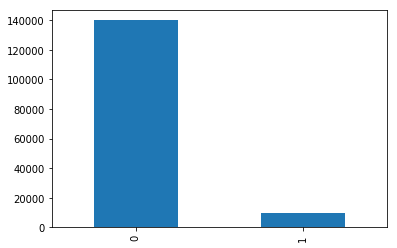In :
df['SeriousDlqin2yrs'].mean()

Out:
0.06684

### Serious delinquincies¶

In :
df['SeriousDlqin2yrs'].describe()

Out:
count    150000.000000
mean          0.066840
std           0.249746
min           0.000000
25%           0.000000
50%           0.000000
75%           0.000000
max           1.000000
Name: SeriousDlqin2yrs, dtype: float64

Nothing odd here.

### Age¶

There's someone aged 0, which should be an error. Let's set it to 21, the minimum age that makes sense. We can also set it to the median age, but this won't make a huge difference considering there are 150,000 observations.

In :
df['age'].value_counts().sort_index().iloc[:20]

Out:
0        1
21     183
22     434
23     641
24     816
25     953
26    1193
27    1338
28    1560
29    1702
30    1937
31    2038
32    2050
33    2239
34    2155
35    2246
36    2379
37    2521
38    2631
39    2987
Name: age, dtype: int64
In :
df['age'].value_counts().sort_index().iloc[-10:]

Out:
96     18
97     17
98      6
99      9
101     3
102     3
103     3
105     1
107     1
109     2
Name: age, dtype: int64
In :
df.loc[df['age']==0,'age'] = 21


### Revolving Utilization Of Unsecured Lines¶

This should be a ratio but for many observations they seem to actually be dollar amounts. Maybe the denominator was 1.

In :
df['RevolvingUtilizationOfUnsecuredLines'].describe()

Out:
count    150000.000000
mean          6.048438
std         249.755371
min           0.000000
25%           0.029867
50%           0.154181
75%           0.559046
max       50708.000000
Name: RevolvingUtilizationOfUnsecuredLines, dtype: float64
In :
df['RevolvingUtilizationOfUnsecuredLines'].median()

Out:
0.154180737
In :
freq=pd.DataFrame(sorted(df['RevolvingUtilizationOfUnsecuredLines']))
freq.quantile(q=np.arange(0.995,0.99999,0.0001)).plot()
plt.title('Revolving Utilization by Percentile')

Out:
Text(0.5,1,'Revolving Utilization by Percentile')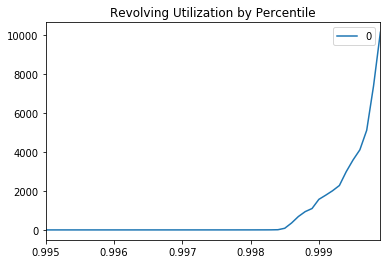The values only start going crazy at some point after the 99.8th percentile, so there fewer than 300 "irregular" values.

In :
freq.quantile(q=[0.998])

Out:
0
0.998 2.761009
In :
df[df['MonthlyIncome']>=2.761009].head()

Out:
SeriousDlqin2yrs RevolvingUtilizationOfUnsecuredLines age NumberOfTime30-59DaysPastDueNotWorse DebtRatio MonthlyIncome NumberOfOpenCreditLinesAndLoans NumberOfTimes90DaysLate NumberRealEstateLoansOrLines NumberOfTime60-89DaysPastDueNotWorse NumberOfDependents
1 1 0.766127 45 2 0.802982 9120.0 13 0 6 0 2.0
2 0 0.957151 40 0 0.121876 2600.0 4 0 0 0 1.0
3 0 0.658180 38 1 0.085113 3042.0 2 1 0 0 0.0
4 0 0.233810 30 0 0.036050 3300.0 5 0 0 0 0.0
5 0 0.907239 49 1 0.024926 63588.0 7 0 1 0 0.0

With a media of 0.154 and mean of 6.04 there is huge positive skewness. It's possible that there is some inconsistency in the data. Even accounts that are victims of fraud will not have rates in the thousands. It is possible that they were recorded as dollar amounts, or when credit card limits were unavailable, 1 was substituted in the denominators.

Regardless, we should deal with this problem in some way, such as censoring all amounts above some threshold. We will play it safe and use 2.5.

In :
df['RevolvingUtilizationOfUnsecuredLines'] = df['RevolvingUtilizationOfUnsecuredLines'].apply(lambda i:i if i <= 2.5 else 2.5)

In :
plt.plot(df['RevolvingUtilizationOfUnsecuredLines'], 'bo', markersize=2)

Out:
[<matplotlib.lines.Line2D at 0x282e085c550>]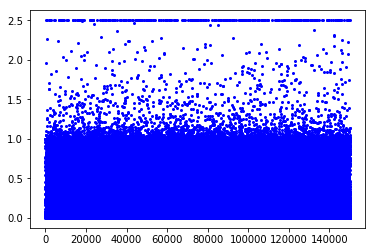There are also other ways to deal with the outliers. For one, we can ignore them, since decision tree models

### Lateness¶

• NumberOfTime30-59DaysPastDueNotWorse
• NumberOfTime60-89DaysPastDueNotWorse
• NumberOfTimes90DaysLate

There are some outliers in these values. While it may be feasible, if unlikely, for someone to have nearly 100 loans past due, in the actual data, these people with huge numbers of defaults don't seem more likely to default than anyone else.

In :
df['NumberOfTime30-59DaysPastDueNotWorse'].value_counts().sort_index()

Out:
0     126018
1      16033
2       4598
3       1754
4        747
5        342
6        140
7         54
8         25
9         12
10         4
11         1
12         2
13         1
96         5
98       264
Name: NumberOfTime30-59DaysPastDueNotWorse, dtype: int64
In :
df['NumberOfTime30-59DaysPastDueNotWorse'] = [v if v <= 13 else np.median(df['NumberOfTime30-59DaysPastDueNotWorse'])
for v in df['NumberOfTime30-59DaysPastDueNotWorse']]

In :
df['NumberOfTime60-89DaysPastDueNotWorse'].value_counts().sort_index()

Out:
0     142396
1       5731
2       1118
3        318
4        105
5         34
6         16
7          9
8          2
9          1
11         1
96         5
98       264
Name: NumberOfTime60-89DaysPastDueNotWorse, dtype: int64
In :
df['NumberOfTime60-89DaysPastDueNotWorse'] = [v if v <= 11 else np.median(df['NumberOfTime60-89DaysPastDueNotWorse'])
for v in df['NumberOfTime60-89DaysPastDueNotWorse']]

In :
df['NumberOfTimes90DaysLate'].value_counts().sort_index()

Out:
0     141662
1       5243
2       1555
3        667
4        291
5        131
6         80
7         38
8         21
9         19
10         8
11         5
12         2
13         4
14         2
15         2
17         1
96         5
98       264
Name: NumberOfTimes90DaysLate, dtype: int64
In :
df['NumberOfTimes90DaysLate'] = [v if v <= 17 else np.median(df['NumberOfTimes90DaysLate'])
for v in df['NumberOfTimes90DaysLate']]

In :
latecor = df[['SeriousDlqin2yrs','NumberOfTime30-59DaysPastDueNotWorse','NumberOfTime60-89DaysPastDueNotWorse','NumberOfTimes90DaysLate']].copy()

In :
for c in latecor.columns:
latecor[c] = [v if v < 1 else 1 for v in latecor[c]]

In :
sns.heatmap(latecor.corr())

Out:
<matplotlib.axes._subplots.AxesSubplot at 0x282e0c25470>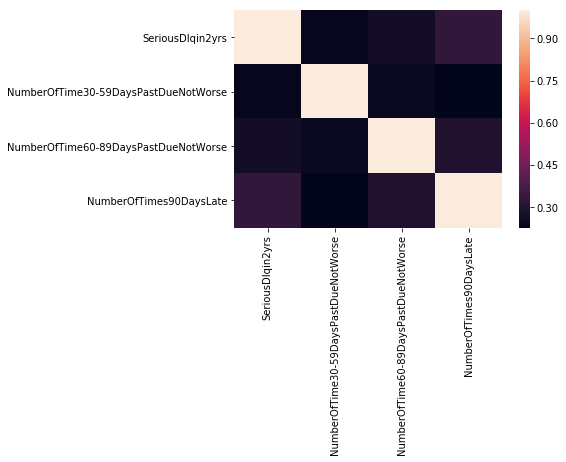In :
latecor.corr()

Out:
SeriousDlqin2yrs NumberOfTime30-59DaysPastDueNotWorse NumberOfTime60-89DaysPastDueNotWorse NumberOfTimes90DaysLate
SeriousDlqin2yrs 1.000000 0.238023 0.263986 0.329598
NumberOfTime30-59DaysPastDueNotWorse 0.238023 1.000000 0.251190 0.227149
NumberOfTime60-89DaysPastDueNotWorse 0.263986 0.251190 1.000000 0.291774
NumberOfTimes90DaysLate 0.329598 0.227149 0.291774 1.000000

We can see that the correlation between delinquincy within 2 years increases in the number of days of lateness.

Past lateness alone is not a very accurate predictor of future delinquency, but they are a valuable contributor. In a linear model, the probability of delinquency conditional on having prior lateness would be higher depending on the degree of past lateness. In a decision tree we can imagine, for example, that a path with a larger number of prior late notices, low income, high debt, and a larger number of dependents would be very likely to have a serious delinquency. Conditional probability is one of the most powerful concepts in the universe.

### Number of dependents¶

There is no reason to suspect any of these numbers, even the 2 outliers within 13 and 20 dependents. Those numbers are not out of the question at all. We can set the 2 outliers to 10, but we'll leave them be for now.

There is missing data we will fill with the median, which turns out to just be 0.

In :
df['NumberOfDependents'].value_counts().sort_index()

Out:
0.0     86902
1.0     26316
2.0     19522
3.0      9483
4.0      2862
5.0       746
6.0       158
7.0        51
8.0        24
9.0         5
10.0        5
13.0        1
20.0        1
Name: NumberOfDependents, dtype: int64
In :
df['NumberOfDependents'].median()

Out:
0.0
In :
df['NumberOfDependents'].fillna(0, inplace=True)


### Real estate loans¶

Like unsecured credit utilization, there are a small number of outliers that we can choose to censor. Let's set 15 as a threshold. These higher numbers of real estate loans don't necessarily give us that much relevant information.

In :
df['NumberRealEstateLoansOrLines'].value_counts().sort_index()

Out:
0     56188
1     52338
2     31522
3      6300
4      2170
5       689
6       320
7       171
8        93
9        78
10       37
11       23
12       18
13       15
14        7
15        7
16        4
17        4
18        2
19        2
20        2
21        1
23        2
25        3
26        1
29        1
32        1
54        1
Name: NumberRealEstateLoansOrLines, dtype: int64
In :
df['NumberRealEstateLoansOrLines'] = [v if v <= 15 else np.median(df['NumberRealEstateLoansOrLines'])
for v in df['NumberRealEstateLoansOrLines']]

In :
df.info()

<class 'pandas.core.frame.DataFrame'>
Int64Index: 150000 entries, 1 to 150000
Data columns (total 11 columns):
SeriousDlqin2yrs                        150000 non-null int64
RevolvingUtilizationOfUnsecuredLines    150000 non-null float64
age                                     150000 non-null int64
NumberOfTime30-59DaysPastDueNotWorse    150000 non-null float64
DebtRatio                               150000 non-null float64
MonthlyIncome                           120269 non-null float64
NumberOfOpenCreditLinesAndLoans         150000 non-null int64
NumberOfTimes90DaysLate                 150000 non-null float64
NumberRealEstateLoansOrLines            150000 non-null float64
NumberOfTime60-89DaysPastDueNotWorse    150000 non-null float64
NumberOfDependents                      150000 non-null float64
dtypes: float64(8), int64(3)
memory usage: 13.7 MB


### Debt Ratio¶

As with revolving utilization, the ratio is not too informative when the denominator is very low. 329664 doesn't make much since as a debt ratio, but it makes a lot of sense for a dollar amount. Indeed, the documentation describes DebtRatio as debt payments, alimony and living costs divided by monthly gross income. We can surmise that when income was 0 or was missing, 1 was substituted.

In :
df['DebtRatio'].describe()

Out:
count    150000.000000
mean        353.005076
std        2037.818523
min           0.000000
25%           0.175074
50%           0.366508
75%           0.868254
max      329664.000000
Name: DebtRatio, dtype: float64
In :
df[df['MonthlyIncome'].isnull()].describe()['DebtRatio']

Out:
count     29731.000000
mean       1673.396556
std        4248.372895
min           0.000000
25%         123.000000
50%        1159.000000
75%        2382.000000
max      329664.000000
Name: DebtRatio, dtype: float64

This actually gives us an interesting opportunity to create a monthly payments feature. But first let's look at the accounts with 1 monthly income. It's very interesting to see that there are significantly fewer delinquincies among people with 1 than those with 0, and fewer even than those with higher incomes. Although this could just mean that those with lower incomes receive fewer loans, and perhaps those with 0 income did not have 0 when the loans were taken.

In :
df['MonthlyIncome'].describe()

Out:
count    1.202690e+05
mean     6.670221e+03
std      1.438467e+04
min      0.000000e+00
25%      3.400000e+03
50%      5.400000e+03
75%      8.249000e+03
max      3.008750e+06
Name: MonthlyIncome, dtype: float64
In :
df['MonthlyIncome'].value_counts().sort_index()[:50]

Out:
0.0     1634
1.0      605
2.0        6
4.0        2
5.0        2
7.0        1
9.0        1
10.0       2
11.0       1
15.0       1
21.0       1
25.0       1
27.0       2
34.0       1
40.0       3
42.0       1
50.0       4
Name: MonthlyIncome, dtype: int64
In :
df[(df['MonthlyIncome']==0)]['SeriousDlqin2yrs'].mean()

Out:
0.04039167686658507
In :
df[(df['MonthlyIncome']==1)]['SeriousDlqin2yrs'].mean()

Out:
0.02809917355371901
In :
df[(df['MonthlyIncome']>=750) & (df['MonthlyIncome']<=1250)]['SeriousDlqin2yrs'].mean()

Out:
0.08394869801787797
In :
df[(df['MonthlyIncome']>=1) & (df['MonthlyIncome']<=15)]['SeriousDlqin2yrs'].mean()

Out:
0.028985507246376812
In :
df[(df['MonthlyIncome']>=16) & (df['MonthlyIncome']<=5400)]['SeriousDlqin2yrs'].mean()

Out:
0.08727178780571822
In :
df[(df['MonthlyIncome']>=5400) & (df['MonthlyIncome']<=8249)]['SeriousDlqin2yrs'].mean()

Out:
0.060808575398663404
In :
df[(df['MonthlyIncome']>=8249)]['SeriousDlqin2yrs'].mean()

Out:
0.046255653099228515

It's probably best to leave these values as they are. While for example 1000 might have mistakenly been entered as 1, overall the non-zero low income observations have very low delinquincy rates, and it would be unwise to make any assumptions. The "1" can mean anything: unknown income, 0 income, people with trust funds, people with non traditional incomes, etc. But they are a very small group (605) with an exceptionall low rate of serious delinquency.

From monthly income and debt ratio, we can determine the monthly payments. This could be extremely valuable, since people with the same debt ratio may be in vastly different economic situations. Imagine 3 cases with a 1.0 debt ratio:

1. \$200 income, \$200 payment
2. \$2000 income, \$2000 payment
3. \$20000 income, \$20000 payment

The first case seems like a college student with a part time job and limited expenses, but who has their room and board covered by their parents. The second case could be a working class person struggling to make ends meet, while the third case could be someone with a high income who is borrowing for investment purposes, has a gambling problem, or got hit with a nasty divorce settlement. Each person has their own distinct challenges when it comes to repaying their loans. Consider a situation where all three lose their jobs: the third person is going to have the most to make up for.

These values can also interact with age: for the first case, the person being 21 or 22 would be a vastly different situation than if it was a 50 year old. In short, the separate dollar amounts can give us different information than the ratios.

In :
payments = []
for i in range(len(df)):
if df['MonthlyIncome'].iloc[i] >= 0:
payments.append(df['MonthlyIncome'].iloc[i] * df['DebtRatio'].iloc[i])
else:
payments.append(df['DebtRatio'].iloc[i])

In :
df['MonthlyPayments']=payments

In :
df.head()

Out:
SeriousDlqin2yrs RevolvingUtilizationOfUnsecuredLines age NumberOfTime30-59DaysPastDueNotWorse DebtRatio MonthlyIncome NumberOfOpenCreditLinesAndLoans NumberOfTimes90DaysLate NumberRealEstateLoansOrLines NumberOfTime60-89DaysPastDueNotWorse NumberOfDependents MonthlyPayments
1 1 0.766127 45 2.0 0.802982 9120.0 13 0.0 6.0 0.0 2.0 7323.197016
2 0 0.957151 40 0.0 0.121876 2600.0 4 0.0 0.0 0.0 1.0 316.878123
3 0 0.658180 38 1.0 0.085113 3042.0 2 1.0 0.0 0.0 0.0 258.914887
4 0 0.233810 30 0.0 0.036050 3300.0 5 0.0 0.0 0.0 0.0 118.963951
5 0 0.907239 49 1.0 0.024926 63588.0 7 0.0 1.0 0.0 0.0 1584.975094
In :
df['MonthlyPayments'].describe()

Out:
count    150000.000000
mean       2029.675384
std        4210.622764
min           0.000000
25%         510.744628
50%        1528.901456
75%        2787.698312
max      478450.559160
Name: MonthlyPayments, dtype: float64
In :
df[df['MonthlyPayments']>250000]

Out:
SeriousDlqin2yrs RevolvingUtilizationOfUnsecuredLines age NumberOfTime30-59DaysPastDueNotWorse DebtRatio MonthlyIncome NumberOfOpenCreditLinesAndLoans NumberOfTimes90DaysLate NumberRealEstateLoansOrLines NumberOfTime60-89DaysPastDueNotWorse NumberOfDependents MonthlyPayments
32535 0 0.067454 76 0.0 44.173674 5993.0 16 0.0 0.0 0.0 1.0 264732.826304
34110 0 0.000000 63 5.0 30.326467 8300.0 16 1.0 2.0 1.0 0.0 251709.673527
36601 0 0.001782 65 0.0 326442.000000 NaN 6 0.0 1.0 0.0 0.0 326442.000000
50217 0 0.340559 63 0.0 28.171455 15000.0 14 0.0 4.0 0.0 1.0 422571.828600
55487 0 0.228582 65 0.0 65.712209 7223.0 14 0.0 0.0 0.0 0.0 474639.287774
59910 1 0.698978 48 0.0 35.440782 13500.0 11 0.0 0.0 0.0 1.0 478450.559160
60153 0 0.000000 65 0.0 329664.000000 NaN 9 0.0 3.0 0.0 0.0 329664.000000
95478 0 0.071065 53 0.0 8.852703 33333.0 8 0.0 0.0 0.0 0.0 295087.147299
127048 0 0.034290 58 1.0 307001.000000 NaN 16 0.0 4.0 0.0 2.0 307001.000000
139095 0 0.514390 44 2.0 28.632287 12487.0 9 0.0 1.0 1.0 3.0 357531.367769

### Monthly Income Prediction¶

We need to estimate the missing values. If we had education data, we could fit a Mincer human capital model. But let's see what we can do with what we have.

Log-wages should be useful (but set the 0s to the lowest non-zero amount, 1, to avoid the log 0 problem). We could also do +1 logs.

In :
df['MonthlyIncome'].describe()

Out:
count    1.202690e+05
mean     6.670221e+03
std      1.438467e+04
min      0.000000e+00
25%      3.400000e+03
50%      5.400000e+03
75%      8.249000e+03
max      3.008750e+06
Name: MonthlyIncome, dtype: float64
In :
df['MonthlyIncome'].value_counts().sort_index()[:20]

Out:
0.0     1634
1.0      605
2.0        6
4.0        2
5.0        2
7.0        1
9.0        1
10.0       2
11.0       1
15.0       1
Name: MonthlyIncome, dtype: int64
In :
# Since log 1 is 0 and we're "setting" all 0 values to 1 anyway, this code is effectively the same as that
# without prematurely filling in the missing values with 0. A win-win-win
# Also do the same to the monthly payments
df['logMI'] = [np.log(v) if v > 0 else v for v in df['MonthlyIncome']]
df['logMP'] = [np.log(v) if v > 0 else v for v in df['MonthlyPayments']]

In :
fig,ax = plt.subplots(1,2,figsize=(12,6))
plt.subplot(1,2,1)
df['logMI'].hist(bins=100)
plt.ylabel('freq')
plt.xlabel('log wage')
plt.title('Log wage histogram')

plt.subplot(1,2,2)
df['logMP'].hist(bins=100)
plt.ylabel('freq')
plt.xlabel('log payment')
plt.title('Log payment histogram')

Out:
Text(0.5,1,'Log payment histogram')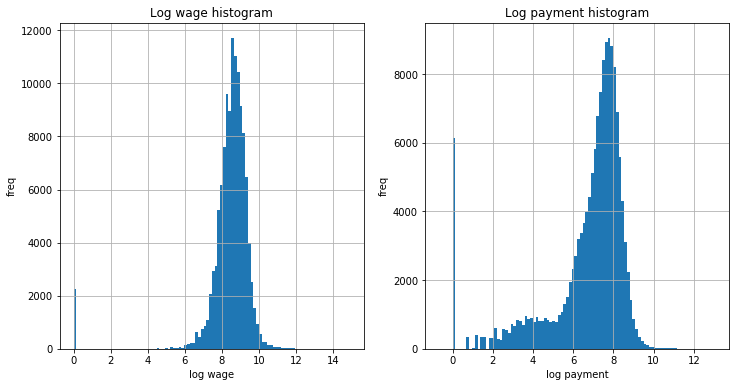Ignoring the 0s, there is something very "normal" looing about log wages, which should not be shocking to anyone in a dataset of this size. Log payment is negatively skewed. In any case, the log transformations make these datum much more manageable.

In :
train = df[df['logMI'].isnull() == False]
test = df[df['logMI'].isnull()]

In :
X_train = train.drop(['MonthlyIncome','logMI','SeriousDlqin2yrs','MonthlyPayments','logMP'], axis=1)
y_train = train['logMI']
X_test = test.drop(['MonthlyIncome','logMI','SeriousDlqin2yrs','MonthlyPayments','logMP'], axis=1)

In :
X_train.head()

Out:
RevolvingUtilizationOfUnsecuredLines age NumberOfTime30-59DaysPastDueNotWorse DebtRatio NumberOfOpenCreditLinesAndLoans NumberOfTimes90DaysLate NumberRealEstateLoansOrLines NumberOfTime60-89DaysPastDueNotWorse NumberOfDependents
1 0.766127 45 2.0 0.802982 13 0.0 6.0 0.0 2.0
2 0.957151 40 0.0 0.121876 4 0.0 0.0 0.0 1.0
3 0.658180 38 1.0 0.085113 2 1.0 0.0 0.0 0.0
4 0.233810 30 0.0 0.036050 5 0.0 0.0 0.0 0.0
5 0.907239 49 1.0 0.024926 7 0.0 1.0 0.0 0.0
In :
grad=GradientBoostingRegressor(learning_rate=0.05,n_estimators=350)

In :
pred = cross_val_predict(grad,X_train,y_train,cv=10)

In :
print(r2_score(y_train,pred))
print(mean_squared_error(y_train,pred))

0.830278507411
0.311218624232


Let's fill in the missing incomes.

In :
grad.fit(X_train,y_train)
test_pred = grad.predict(X_test)

In :
df.loc[df['logMI'].isnull(),'logMI'] = test_pred

In :
df.info()

<class 'pandas.core.frame.DataFrame'>
Int64Index: 150000 entries, 1 to 150000
Data columns (total 14 columns):
SeriousDlqin2yrs                        150000 non-null int64
RevolvingUtilizationOfUnsecuredLines    150000 non-null float64
age                                     150000 non-null int64
NumberOfTime30-59DaysPastDueNotWorse    150000 non-null float64
DebtRatio                               150000 non-null float64
MonthlyIncome                           120269 non-null float64
NumberOfOpenCreditLinesAndLoans         150000 non-null int64
NumberOfTimes90DaysLate                 150000 non-null float64
NumberRealEstateLoansOrLines            150000 non-null float64
NumberOfTime60-89DaysPastDueNotWorse    150000 non-null float64
NumberOfDependents                      150000 non-null float64
MonthlyPayments                         150000 non-null float64
logMI                                   150000 non-null float64
logMP                                   150000 non-null float64
dtypes: float64(11), int64(3)
memory usage: 17.2 MB

In :
df.to_csv('cs-trainmod.csv')

In :
features = ['RevolvingUtilizationOfUnsecuredLines','age','NumberOfTime30-59DaysPastDueNotWorse',
'NumberOfOpenCreditLinesAndLoans','NumberOfTimes90DaysLate','NumberRealEstateLoansOrLines',
'NumberOfTime60-89DaysPastDueNotWorse','NumberOfDependents','logMI','logMP']


## t-SNE and PCA Visualization¶

Let's transform n dimensions into 2. t-SNE emphasizes placing similar features near each other, as opposed to methods like PCA which focus on maximizing variance/separating differences. We can see that t-SNE will cluster a large number of features related to delinquency together.

In :
X = df[features]
y = df['SeriousDlqin2yrs']

sns.set(rc={'figure.figsize':(12,12)})


### t-SNE¶

In :
tsne = TSNE(early_exaggeration=4.0, learning_rate=25.0, n_iter=250)

# this stuff takes a long time, let's take a smaller sample

X_samp, X_notused, y_samp, y_notused = train_test_split(X, y, test_size=0.33)

transtsne = tsne.fit_transform(X_samp)

tsnedf = pd.DataFrame(transtsne, columns=['1', '2'])
y_samp.index = range(0,len(tsnedf))
tsnedf['class'] = y_samp

sns.lmplot(x='1', y='2', data = tsnedf,hue='class',size=10,palette="Set2",fit_reg=False,scatter_kws={'alpha':0.2})

Out:
<seaborn.axisgrid.FacetGrid at 0x28286b6f198>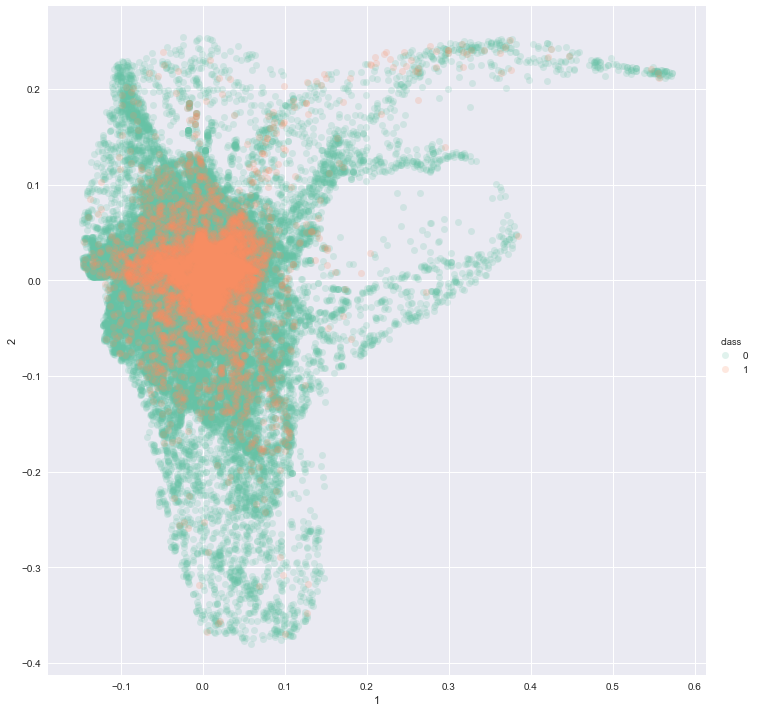### PCA¶

In :
ss = StandardScaler()
X_norm = ss.fit_transform(X)
forPca = pd.DataFrame(X)

pct = PCA(n_components=2)
X_trans = pct.fit_transform(X)
pcadf = pd.DataFrame(X_trans,columns=['1','2'])
pcadf['class'] = y

sns.lmplot(x='1',y='2',data=pcadf,hue='class',size=10,fit_reg=False,palette="Set2",scatter_kws={'alpha':0.2})
#plt.axis([-500, 1500, -50, 50])

Out:
<seaborn.axisgrid.FacetGrid at 0x282e36ee9e8>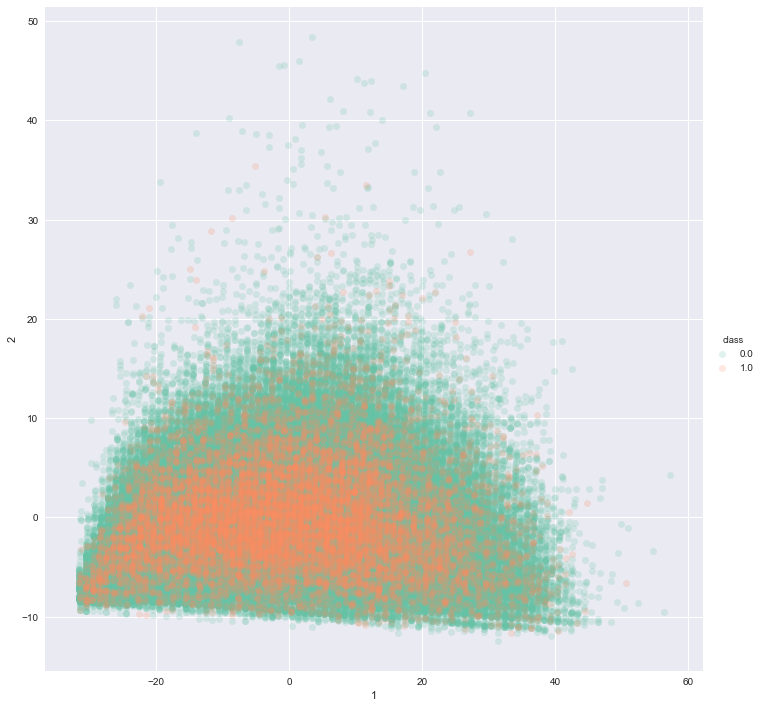We can see that there are significant overlaps, but there are regions where there are clearly more class 0s than 1s. Particularly in the t-SNE graph, there is a major high risk concentration near the center of the cloud.

## Building the model¶

In :
X_train, X_test, y_train, y_test = train_test_split(X, y, test_size=0.2)


As is customary, let's form a linear baseline with logistic regression.

In :
lr = LogisticRegression()
lr.fit(X_train,y_train)
log_pred = lr.predict_proba(X_test)[:,1]
print ('Logistic regression test AUC')
roc_auc_score(y_test,log_pred)

Logistic regression test AUC

Out:
0.85421480295896113
In :
print('Logistic regression training AUC')
roc_auc_score(y_train, lr.predict_proba(X_train)[:,1])

Logistic regression training AUC

Out:
0.84981861970157468
In :
rf = RandomForestClassifier(n_estimators=20)
rf.fit(X_train,y_train)
rf_pred = rf.predict_proba(X_test)[:,1]
print ('Random forest test AUC')
roc_auc_score(y_test,rf_pred)

Random forest test AUC

Out:
0.81093306111327879
In :
print('Random forest training AUC')
roc_auc_score(y_train, rf.predict_proba(X_train)[:,1])

Random forest training AUC

Out:
0.99989903500052468

We can see that logistic regression performs noticeably better on the test set than random forest. It is our experience that in many binary classification problems - especially in credit risk problems - random forest severely overfits on the training set. In fact there usually exists some significantly high number of trees (n_estimators) such that random forest can achieve 100% training set accuracy. Of course, such a model would not be a good predictor.

### Optimize the hyperparameters¶

Maybe get some pizza and watch a movie.

In :
grad_test = GradientBoostingRegressor(learning_rate=0.05)
hyper_params = {'n_estimators': randint(10, 650),
'max_depth': randint(1,10),
'min_samples_split': randint(2,5),
'min_samples_leaf': randint(1,5)}

In :
search = RandomizedSearchCV(grad_test, param_distributions=hyper_params, n_jobs=4, scoring='roc_auc', verbose=4, cv=5)
search.fit(X_train,y_train)
search.best_params_, search.best_score_

Fitting 5 folds for each of 10 candidates, totalling 50 fits

[Parallel(n_jobs=4)]: Done  17 tasks      | elapsed:  3.4min
[Parallel(n_jobs=4)]: Done  50 out of  50 | elapsed: 10.9min finished

Out:
({'max_depth': 6,
'min_samples_leaf': 3,
'min_samples_split': 4,
'n_estimators': 135},
0.86207848826031319)
In :
probs = search.best_estimator_.predict(X_test)

In :
roc_auc_score(y_test,probs)

Out:
0.86955550384248048

USing this method on the test set, we scored 0.866752 on the Kaggle private set (70% of the test set), compared to the 0.869558 first place score.

The numbers seem close, but this disparity could mean several hundred more wrong lending decisions out of 150,000.

In :
xg = xgb.XGBRegressor(learning_rate=0.01, n_jobs=4, eval_metric='mae')
hyper_params1 = {'n_estimators': randint(1000, 2000),
'max_depth': randint(1,12),
'subsample': uniform(0,1),
'min_child_weight': randint(1,10),
'max_delta_step': randint(1,10)}

search1 = RandomizedSearchCV(xg, param_distributions=hyper_params1 ,n_jobs=4, verbose=4, cv=5)
search1.fit(X_train,y_train)
search1.best_params_, search.best_score_

Fitting 5 folds for each of 10 candidates, totalling 50 fits

[Parallel(n_jobs=4)]: Done  17 tasks      | elapsed: 11.3min
[Parallel(n_jobs=4)]: Done  50 out of  50 | elapsed: 22.1min finished

Out:
({'max_delta_step': 9,
'max_depth': 4,
'min_child_weight': 4,
'n_estimators': 1259,
'subsample': 0.55139850235400301},
0.86207848826031319)
In :
probs1 = search1.best_estimator_.predict(X_test)
roc_auc_score(y_test,probs1)

Out:
0.8700812411291321

### Optimal Risk Threshold¶

Let's try to set an optimal risk threshold for a lender, since lending to someone with a slightly below 50% chance of default is probably not a great idea. We will stick with the gradient boosted model since that's what we ultimately used for our Kaggle submission.

In :
highest_auc = 0
opt_thresh = 0
n = 10000
for i in range(n):
thresh = i/n
dec = [1 if p > thresh else 0 for p in probs]
auc = roc_auc_score(y_test,dec)
if auc > highest_auc:
highest_auc = auc
opt_thresh = thresh
print (opt_thresh)
dec = [1 if p > opt_thresh else 0 for p in probs]

0.0723


Only lend when there is less than 7.2 % probability of default.

Compared to simply giving everyone a loan, we deny 1547 bad borrowers at the cost of denying 5381 good borrowers. With more data, it would be possible to go further and estimate the present values of these loans to predict a monetary value for a particular lending scheme. It is reasonable to assume that the average amount lost from a delinquincy greatly exceeds the discounted profit from a good loan.

In :
conf = confusion_matrix(y_test,dec)

norm_conf = conf.astype('float') / conf.sum(axis=1)[:,np.newaxis]

print ('Confusion matrix\n')
print (conf)
print ('\nNormalized confusion matrix\n')
print (norm_conf)
print ('\nClassification report\n')
print (classification_report(y_test,dec))

plt.figure(figsize=(8,8))
sns.heatmap(norm_conf)
plt.title('Confusion heatmap')
plt.ylabel('True classification')
plt.xlabel('Lender classification')

Confusion matrix

[[22632  5381]
[  440  1547]]

Normalized confusion matrix

[[ 0.80791061  0.19208939]
[ 0.22143936  0.77856064]]

Classification report

precision    recall  f1-score   support

0       0.98      0.81      0.89     28013
1       0.22      0.78      0.35      1987

avg / total       0.93      0.81      0.85     30000


Out:
Text(0.5,52,'Lender classification')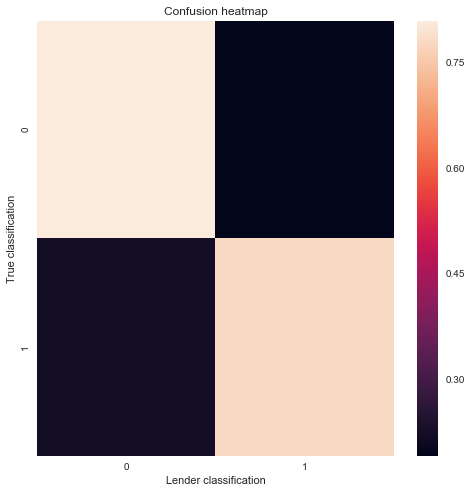## How Useful is Age?¶

Age is useful in income prediction, but how good is it assessing risk in this data set? Should we try to create a model with binned ages or leave it as is for now?

### Weight of Evidence and Information Value¶

We can use a more traditional method such as weight of evidence (WoE) to assess age.

WoE: log(%non event/% event) where the "event" in question is delinquincy

Age is technically already binned, since time is a continuous variable and we're rounding down to whole numbers. Still, grouping them further may make it more useful. As we saw from the income analysis above, there is evidence that income groups can be usefully binned. However, we will stick with age for now.

In :
plt.figure(figsize=(8,8))
df['age'].hist(bins=100)
plt.title('Number of loans by age')

Out:
Text(0.5,1,'Number of loans by age')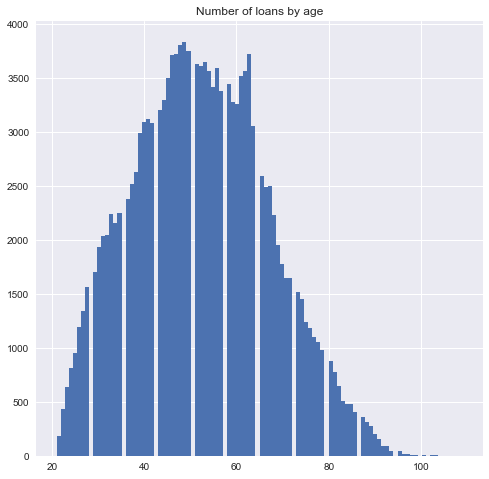Let's be generous and say that everyone above 81 is now 81.

In :
df2 = df.copy()
df2['age'] = [v if v <= 81 else 81 for v in df2['age']]

In :
unique_ages = df2['age'].value_counts().index.sort_values()

In :
bad_age = []
good_age = []
for a in unique_ages:
good_age.append(df2[df2['age']==a].groupby('SeriousDlqin2yrs').count()['age'])
bad_age.append(df2[df2['age']==a].groupby('SeriousDlqin2yrs').count()['age'])

In :
age_df = pd.DataFrame(list(zip(unique_ages,good_age,bad_age)),columns=['Age','TotalNonDlq','TotalDlq'])
total_good = sum(age_df['TotalNonDlq'])
total_dlq = sum(age_df['TotalDlq'])

In :
age_df['PercentNonDlq'] = age_df['TotalNonDlq'].apply(lambda v:v/total_good)
age_df['PercentDlq'] = age_df['TotalDlq'].apply(lambda v:v/total_dlq)

In :
age_df['WoE'] = np.log(age_df['PercentNonDlq']/age_df['PercentDlq'])

In :
plt.figure(figsize=(8,8))
sns.regplot('Age','WoE',age_df,order=3)
plt.title ('WoE by Age')

Out:
Text(0.5,1,'WoE by Age')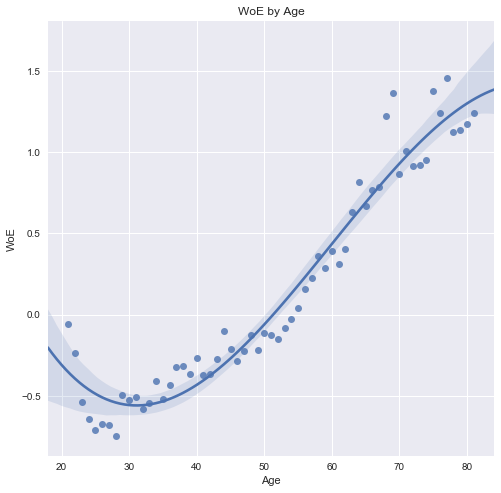The cubic fit line doesn't really have any use, it just looks nice.

We can also find the information value for each age.

In :
age_df['IV'] = age_df['WoE']*(age_df['PercentNonDlq'] - age_df['PercentDlq'])
age_df.head()

Out:
Age TotalNonDlq TotalDlq PercentNonDlq PercentDlq WoE IV
0 21 171 13 0.001222 0.001297 -0.059561 0.000004
1 22 398 36 0.002843 0.003591 -0.233342 0.000174
2 23 571 70 0.004079 0.006982 -0.537381 0.001560
3 24 718 98 0.005130 0.009775 -0.644773 0.002995
4 25 832 121 0.005944 0.012069 -0.708233 0.004338

Siddiqi (2006) suggests that a bin with an IV of under 0.1 has weak predictive power, and under 0.02 it's not useful at all. We should aim for the (0.3,0.5) range.

In :
plt.figure(figsize=[8,8])
age_df['IV'].plot()

Out:
<matplotlib.axes._subplots.AxesSubplot at 0x28286b84be0>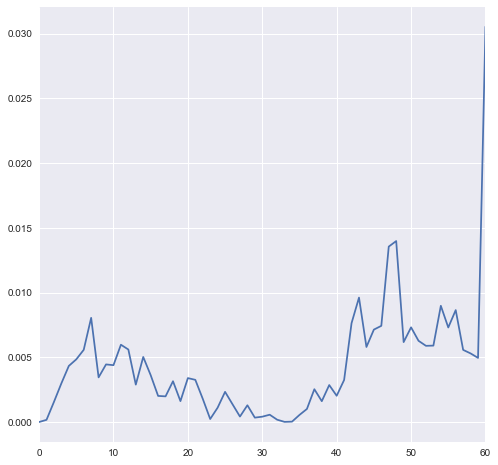The IVs are very low, so let's try binning them further.

In :
freq=pd.DataFrame(sorted(df2['age']))

In :
df2['ageCat'] = np.digitize(np.array(df2['age']),freq.quantile(q=np.arange(0.1,0.9,0.1)).values)

In :
unique_cats = df2['ageCat'].value_counts().index.sort_values()

In :
bad_cat = []
good_cat = []
for a in unique_cats:
good_cat.append(df2[df2['ageCat']==a].groupby('SeriousDlqin2yrs').count()['ageCat'])
bad_cat.append(df2[df2['ageCat']==a].groupby('SeriousDlqin2yrs').count()['ageCat'])

In :
cat_df = pd.DataFrame(list(zip(unique_cats,good_cat,bad_cat)),columns=['ageCat','TotalNonDlq','TotalDlq'])

In :
cat_df['PercentNonDlq'] = cat_df['TotalNonDlq'].apply(lambda v:v/total_good)
cat_df['PercentDlq'] = cat_df['TotalDlq'].apply(lambda v:v/total_dlq)
cat_df['WoE'] = np.log(cat_df['PercentNonDlq']/cat_df['PercentDlq'])
cat_df['IV'] = cat_df['WoE']*(cat_df['PercentNonDlq'] - cat_df['PercentDlq'])

In :
cat_df

Out:
ageCat TotalNonDlq TotalDlq PercentNonDlq PercentDlq WoE IV
0 0 13152 1694 0.093960 0.168961 -0.586794 0.044010
1 1 12775 1396 0.091267 0.139238 -0.422396 0.020263
2 2 14089 1403 0.100654 0.139936 -0.329493 0.012943
3 3 13073 1156 0.093396 0.115300 -0.210692 0.004615
4 4 13872 1151 0.099104 0.114802 -0.147034 0.002308
5 5 13229 1005 0.094510 0.100239 -0.058851 0.000337
6 6 16074 871 0.114836 0.086874 0.279041 0.007802
7 7 13299 568 0.095011 0.056653 0.517048 0.019833
8 8 30411 782 0.217262 0.077997 1.024430 0.142667

It seems like age by itself is not worth binning, with such low IV scores. However, these only apply for age categories on their own. It is entirely possible that they can be the basis for features that may be useful. A few that come to mind include finding the log difference between an individual's income and their age group average, or a similar measure of dependents relative to the group average.

Measures such as these may give additional information about an individual's economic situation and ability to pay, and they would be useful to explore in any further research.

## Conclusion¶

In terms of the baseline unused models, logistic regression performs very well. This is not very surprising, as it is the traditional method of risk classification, with nearly 100 years of theoretical development and practical use. It is also a deterministic model that requires relatively little computing power, and so it can easily and quickly be reproduced on even a mobile device. Being deterministic, these results are 100% consistent for any given set of observations and features. However, on average, the results from boosting methods are clearly superior. Without using XGBoost or more ensemble methods, we managed to achieve a score of 0.866752 on the Kaggle private leaderboard with just a randomized cross-validated gradient boosting model.

The log transformations removed many of the outliers, but it's not clear how much of a difference this made in our final model, since decision tree methods tend to be robust for monotonic transformations (since they are order-preserving). However, they did make the data more palatable and easier to visualize. In addition to the features mentioned above for age we could try traditional methods like including squared features or trying different interactions and ratios between the existing features. We could also try to improve monthly income estimation, which is in itself a large topic. Finding more uses for age could be a major component of that as well.

The prize winning team used over 20 features and was developed over the course of several months. Intellectually, it would have been fascinating to have been a fly on the wall during their deliberations. But in a more practical sense, we have to consider the tradeoff between time and money, and the diminishing returns from spending a lot more time to make relatively minor improvements in the model.